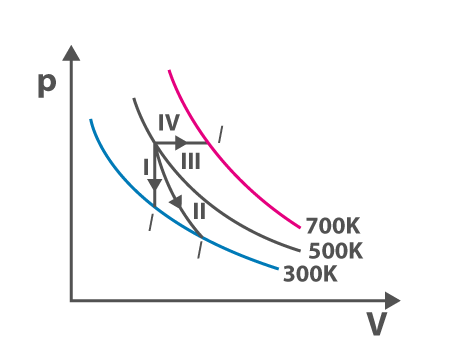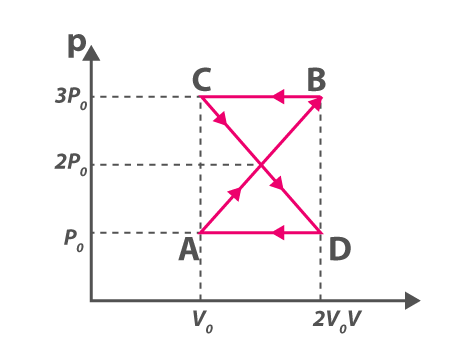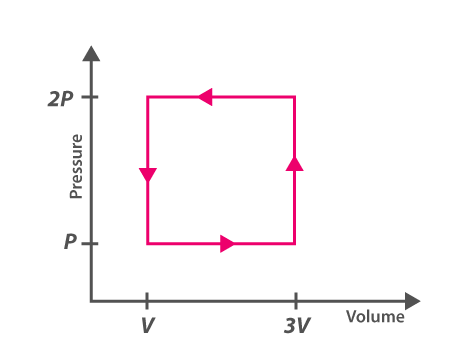# Physics Thermodynamics MCQs for NEET

Thermodynamics is a branch of Physics that helps in understanding heat and temperature and their relation with work, energy, radiation, and other properties of matter.There are four laws of thermodynamics and they are:

• Zeroth law of thermodynamics: The laws state that when two systems are in thermal equilibrium with a third system, then the first two systems are in thermal equilibrium with each other.
• The first law of thermodynamics: Energy can neither be created nor destroyed. It can only be transformed from one form to another.
• The second law of thermodynamics: The entropy of an isolated system that is not in equilibrium will increase over time and reaches the maximum value at equilibrium.
• Third law of thermodynamics: For entropy to approach the constant, the temperature must approach absolute zero.
1. Following is the diagram of the thermodynamic process. Refer the diagram and match the followingP Process – I (a) Adiabatic

Q Process – II (b) Isobaric

R Process – III (c) Isochoric

S Process – IV (d) Isothermal

(a) (P,c), (Q,d), (R,b), (S,a)

(b) (P,c), (Q,d), (R,b), (S,a)

(c) (P,a), (Q,c), (R,d), (S,b)

(d) (P,c), (Q,a), (R,d), (S,b)

Answer: (d) (P,c), (Q,a), (R,d), (S,b)

2. Which of the following process is used to do maximum work done on the ideal gas if the gas is compressed to half of its initial volume?

(a) Isothermal

(b) Isochoric

(c) Isobaric

3. ABCDA is a cyclic process explaining the thermodynamic process. What is the work done by the system in the cycle(a) Zero

(b) $$\frac{\rho _{0}V_{0}}{2}$$

(c) ρ0V0

(d) 2ρ0V0

4. What is the ratio of $$\frac{C_{p}}{C_{v}}$$ for gas if the pressure of the gas is proportional to the cube of its temperature and the process is an adiabatic process.

(a) 2

(b) 3/2

(c) 4/3

(d) 5/3

5. The coefficient performance of a refrigerator is 5. If the temperature inside the freezer is -20℃, calculate the heat rejected to the surrounding

(a) 11℃

(b) 41℃

(c) 21℃

(d) 31℃

6. A thermodynamic system is explained with the help of the graph ABCD. What is the heat rejected by the system during the cycle?(a) pV

(b) 2pV

(c) 4pV

(d) 1/2pV

7. Select the incorrect statement

(a) For the triple point of water, 1°K is equal to 1/273.16.

(b) The first law of thermodynamics is also known as the law of thermal equilibrium

(c) The triple point of water is one of the reference points on the thermodynamic scale of temperature

8. What is a necessary condition for a reaction to be spontaneous at all temperatures?

(a) ΔH < ΔG

(b) ΔG and ΔH should be positive

(c) ΔH = ΔG = 0

(d) ΔG and ΔH should be negative

Answer: (d) ΔG and ΔH should be negative

9. A gas is compressed to half of its initial volume isothermally. The same gas is compressed again until the volume reduces to half through an adiabatic process. Then

(a) Work done during isothermal compression is more

(b) Work done is independent of the processes used for compression

(c) Work done is more during the adiabatic process

(d) Work done is dependent on the atomicity of the gas

10. Select the factor that affects the heat of reaction which is based on Kirchoff’s equation

(a) Molecularity

(b) Temperature

(c) Pressure

(d) Volume

11. For all the reactions what is the nature of the chemical dissociation?

(a) Exothermic

(b) Reversible

(c) Endothermic

(d) Reversible and endothermic

12.Which of the following is the largest unit of energy

(a) Electron volt

(b) Joule

(c) Calorie

(d) Erg